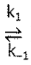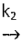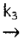# Problem: The proposed mechanism for a given reaction is as follows:Step One: 2 NO (g)    N2O2 (g)Step Two: H2(g) + N2O2(g)    H2O(g) + N2O(g)      Rate - determining StepStep Three: N2O(g) + H2(g)    N2(g) + H2O(g)    FastUnder what conditions would this reaction be first-order in H2? Please answer and justify your reasoning in 1-2 complete sentences on the line provided.

###### FREE Expert Solution

For this problem, we are being asked on what conditions would this reaction be first order in H2.

In this case, we have to revisit the steady-state approximation for the intermediates

Recall that the rate law only focuses on the reactant concentrations and has a general form of:

k = rate constant
A & B = reactants
x & y = reactant orders

• A steady-state approach makes use of the assumption that the rate of production of an intermediate is equal to the rate of its consumption.
• We will be starting this with:

98% (270 ratings)###### Problem Details

The proposed mechanism for a given reaction is as follows:

Step One: 2 NO (g)N2O2 (g)

Step Two: H2(g) + N2O2(g)H2O(g) + N2O(g)      Rate - determining Step

Step Three: N2O(g) + H2(g)N2(g) + H2O(g)    Fast

Under what conditions would this reaction be first-order in H2? Please answer and justify your reasoning in 1-2 complete sentences on the line provided.